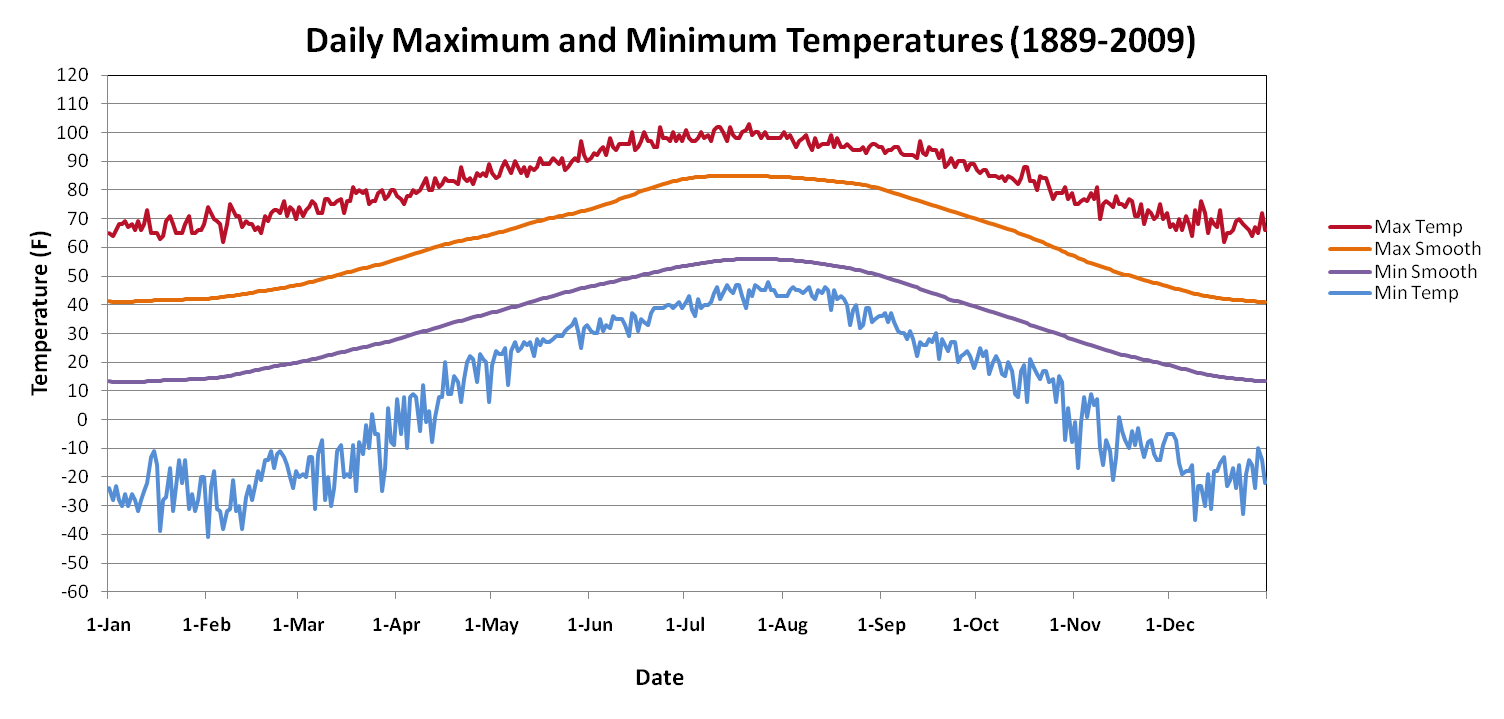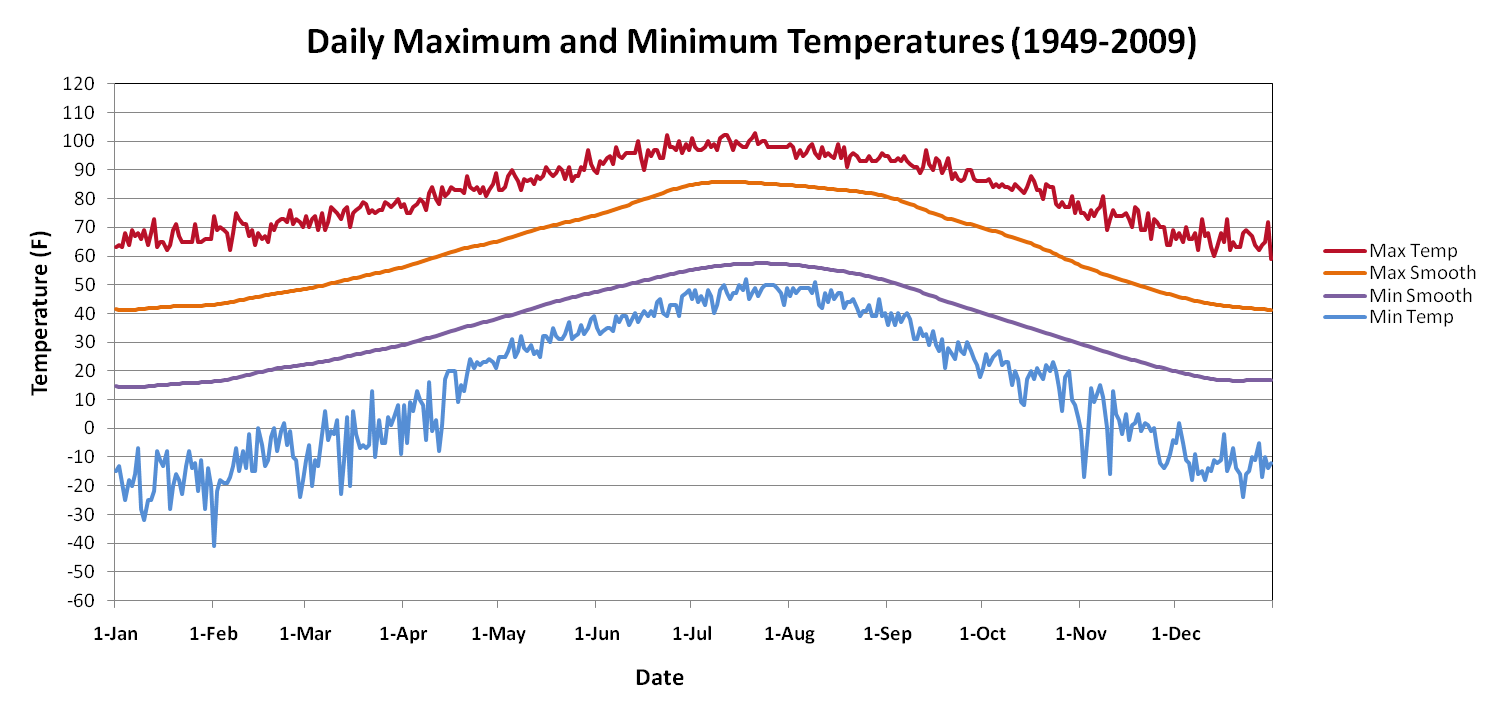Data was collected from the Fort Collins Weather Station archive and graphed over two differing time spans. The first plot describes the daily maximum and minimum temperatures over a period of 120 years from 1889-2009 while the second plot describes the daily maximum and minimum temperatures over a period of 60 years from 1949-2009. For each graph, the daily maximum and minimum temperatures are the record high and low temperatures measured on their respective date. For example, the highest temperature ever recorded for June 1st from the time period of 1889-2009 was 91 degrees Fahrenheit in 1940 while the coolest temperature ever recorded for that date was 31 degrees Fahrenheit in 1922. In comparison, the second graph which spans from 1949-2009 has a record high of 89 degrees Fahrenheit for June 1, 1972 and a record low of 25 degrees Fahrenheit in 1951 .

To achieve the smoothed curve measurement the daily maximum and minimum temperatures for each date were first averaged providing a single average maximum for each date and a single average minimum for each date. June 1, for example, has a daily maximum average from 1889-2009 of 72.64 degrees Fahrenheit and a minimum average of 46.34 degrees Fahrenheit. To this data, a Gaussian filter was applied with an e-folding length of 31 days to isolate the seasonal cycle. In order to apply this equation to a date such as January 1st that does not have 31 dates before it to utilize for smoothing, the average temperatures from December were utilized in the equation. The same technique was used for the end of the year where January temperatures were used to smooth daily December temperatures.

Distribution values ranging from 0-31 were squared and then multiplied by -0.01 . This value was then applied in an exponential function and this determined the weight to be applied to the 31 days both before and after the single date being smoothed around.